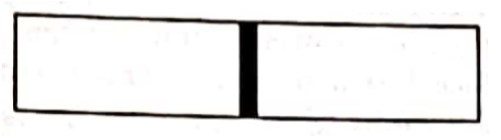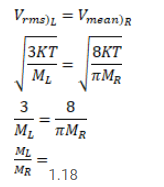# Use R=8.3 J/mol-K wherever required. Figure shows a vessel partitioned by a fixed diathermic separator.Question:

Use $\mathrm{R}=8.3 \mathrm{~J} / \mathrm{mol}-\mathrm{K}$ wherever required.

Figure shows a vessel partitioned by a fixed diathermic separator. Different ideal gases are filled in the two parts. The rms speed of the molecules in the left part equals the mean speed of the molecules in the right. Calculate the ratio of the mass of a molecule molecule in the left part of the mass of a molecule in the right part.Solution: